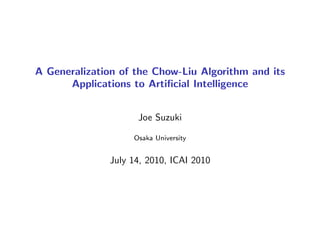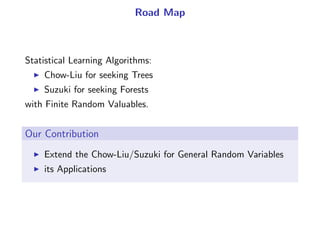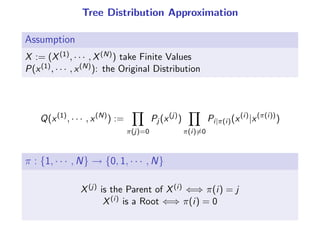Successfully reported this slideshow.

# A Generalization of the Chow-Liu Algorithm and its Applications to Artificial Intelligence

0

Share×
1 of 18
1 of 18

# A Generalization of the Chow-Liu Algorithm and its Applications to Artificial Intelligence

0

Share

A Generalization of the Chow-Liu Algorithm and its
Applications to Artificial Intelligence, Joe Suzuki
Osaka University, July 14, 2010, ICAI 2010

A Generalization of the Chow-Liu Algorithm and its
Applications to Artificial Intelligence, Joe Suzuki
Osaka University, July 14, 2010, ICAI 2010

## More Related Content

### Related Books

Free with a 14 day trial from Scribd

See all

### Related Audiobooks

Free with a 14 day trial from Scribd

See all

### A Generalization of the Chow-Liu Algorithm and its Applications to Artificial Intelligence

1. 1. A Generalization of the Chow-Liu Algorithm and its Applications to Artiﬁcial Intelligence Joe Suzuki Osaka University July 14, 2010, ICAI 2010
2. 2. Road Map Statistical Learning Algorithms: Chow-Liu for seeking Trees Suzuki for seeking Forests with Finite Random Valuables.   Our Contribution Extend the Chow-Liu/Suzuki for General Random Variables its Applications
3. 3. Tree Distribution Approximation Assumption X := (X(1), · · · , X(N)) take Finite Values P(x(1), · · · , x(N)): the Original Distribution Q(x(1) , · · · , x(N) ) := ∏ π(j)=0 Pj (x(j) ) ∏ π(i)̸=0 Pi|π(i)(x(i) |x(π(i)) ) π : {1, · · · , N} → {0, 1, · · · , N} X(j) is the Parent of X(i) ⇐⇒ π(i) = j X(i) is a Root ⇐⇒ π(i) = 0
4. 4. Example Q(x(1) , x(2) , x(3) , x(4) ) = P1(x(1) )P2(x(2) |x(1) )P3(x(3) |x(2) )P4(x(4) |x(2) ) X(1) X(2) X(3) X(4) E E T π(1) = 0 , π(2) = 1 , π(3) = 2 , π(4) = 2
5. 5. Kullback-Leibler and Mutual Information Kullback-Leibler Information D(P||Q) := ∑ x(1),··· ,x(N) P(x(1) , · · · , x(N) ) log P(x(1), · · · , x(N)) Q(x(1), · · · , x(N)) (distribution diﬀerence) Mutual Infomation I(X, Y ) := ∑ x,y PXY (x, y) log PXY (x, y) PX (x)PY (y) (correlation)
6. 6. The Chow-Liu Algorithm P: the Original Q: its Tree Approximation We wish to ﬁnd Q s.t. D(P||Q) → Min Find such Parents (π(1), · · · , π(N)) Chow-Liu, 1968 Continue to select an edge (X(i), X(j)) s.t. I(X(i), X(j)) → Max unless adding it makes a Loop.
7. 7. Example i 1 1 2 1 2 3 j 2 3 3 4 4 4 I(i, j) 12 10 8 6 4 2 1. I(1, 2): Max =⇒ Connect X(1), X(2). 2. I(1, 3): Max except above =⇒ Connect X(1), X(3). 3. The connection (2, 3): will make a Loop. 4. I(1, 4): Max except above =⇒ Connect X(1), X(4) 5. Any further connection will make a Loop.
8. 8. X(2) X(4) X(1) X(3)
9. 9. X(2) X(4) X(1) X(3)
10. 10. X(2) X(4) X(1) X(3)
11. 11. X(2) X(4) X(1) X(3) d dd
12. 12. Chow-Liu: the Procedure V = {1, · · · , N} I(i, j) := I(X(i), X(j)) (i ̸= j) 1. E := {}; 2. E := {{i, j}|i ̸= j}; 3. for {i, j} ∈ E maximizing Ii,j , E := E{{i, j}}; 4. For (V , E ∪ {{i, j}}) not containing a loop: E := E ∪ {{i, j}}; 5. If E ̸= {}, go to 3. and terminate otherwise; Chow-Liu gives the Optimal (mathematically proved). Q expressed by G = (V , E) minimizes D(P||Q).
13. 13. The Chow-Liu Algorithm for Learning Only n examples are given xn := {(x (1) i , · · · , x (N) i )}n i=1 Use Empirical MI: In(i, j) = 1 n ∑ x,y ci,j (x, y) log ci,j (x, y) ci (x)cj (y) ci,j (x, y), ci (x), cj (y): Frequencies in xn   Seeking only a Tree Seeking a Forest as well as a Tree (Suzuki, UAI-93): use Jn(i, j) := In(i, j) − 1 2 (α(i) − 1)(α(j) − 1) log n Stop when Jn(i, j) 0. α(i): How many values X(i) takes.
14. 14. Suzuki UAI-93 i j In(i, j) α(i) α(j) Jn(i, j) 1 2 12 5 2 8 1 3 10 5 3 2 2 3 8 2 3 6 1 4 6 5 4 -6 2 4 4 2 4 1 3 4 2 3 4 -4 1. Jn(1, 2) = 8: Max =⇒ Connect X(1), X(2). 2. Jn(2, 3) = 6: Max except above =⇒ Connect X(2), X(3). 3. Connecting X(1), X(3) will make a Loop. 4. Jn(2, 4) = 1: Max except above =⇒ Connect X(2), X(4). 5. For the rest, Jn 0 or making a Loop.
15. 15. X(2) X(4) X(1) X(3)
16. 16. X(2) X(4) X(1) X(3)
17. 17. X(2) X(1) X(4) X(3)
18. 18. X(2) X(4) X(1) X(3)
19. 19. Modiﬁcation Base on the Minimum Descripion Length Jn(i, j) := In(i, j) − 1 2 (α(i) − 1)(α(j) − 1) log n Generating a forest rather than a tree (Stop when Jn 0). Balancing the data ﬁtness the forest complexity by connecting or not connecting each of the edges The Suzuki minimizes the DL (mathematically proven). H(xn |π) + k(π) 2 log n → min π = (π(1), · · · , π(N)): Parents H(xn|π): (−1)× Likelihood of xn given π k(π): # of Parameters in π
20. 20. Discrete and Continuous: rather Special Cases X = −1 with Prob. 1/2 X = x ≥ 0 with Prob. 1/2 FX (x) =    0 x −1 1 2 1 ≤ x 0 1 2 ∫ x 0 g(t)dt 0 ≤ x ( ∫ ∞ 0 g(x)dx = 1) No Density Function fX for the FX (x) = ∫ x −∞ fX (t)dt.
21. 21. General Random Variables (Ω, F, µ): Probability Space B: the Borel Set Field of R X : Ω → R is a Random Variable in (Ω, F, µ) D ∈ B =⇒ {ω ∈ Ω|X(ω) ∈ D} ∈ F µX : B → R is the Probability Measure of X D ∈ B =⇒ µX (D) := µ({ω ∈ Ω|X(ω) ∈ D})
22. 22. Kullback-Leibler and Mutual Information Kullback-Leibler Information If µ ν, D(µ||ν) := ∫ Ω dµ log dµ dν dµ dν := f s.t. µ = ∫ fdν (Radon-Nikodym) Mutual Info. I(X, Y ) := ∫ Ω dµXY log d2µXY dµX dµY dµXY dµX dµY := g s.t. µXY = ∫ gdµX dµY (Radon-Nikodym)
23. 23. Chow-Liu for General Random Variables Tree Approximation: for D1, · · · , DN ∈ B, ν(D1, · · · , DN) = ∏ π(i)̸=0 µi,π(i)(Di , Dπ(i)) µi (Di )µπ(i)(Dπ(i)) · N∏ i=1 µi (Di ) Theorem The Chow-Liu works even for General Random Variables Proof Sketch: D(µ||ν) = − ∑ π(i)̸=0 I(X(i) , X(π(i)) )+(Const.)
24. 24. Example 1: Multivariate Gaussian Distributions X(i) ∼ N(0, σ2) (X(i) , X(j) ) ∼ N(0, Σ), Σ = [ σii σij σji σjj ] , ρij := σij √ σii σjj I(i, j) = − 1 2 log (1 − ρij 2 ) In(i, j) := − 1 2 log (1 − ˆρij 2 ) Jn(i, j) := In(i, j) − 1 2 log n L(π, xn ) = − ∑ π(i)̸=0 Jn(i, π(i)) + (Const.) Maximizing Jn leads to minimizing DL.
25. 25. Example 2: Gaussian and Finite-Value Random Variables X(i): Gaussian X(j): takes α(j) values I(i, j) = ∑ y∈X(j) µj (y) ∫ x∈X(i) fi,j (x|y) log fi,j (x|y) ∑ z∈X(j) µj (z)fi,j (x|z) dx Jn(i, j) := In(i, j) − α(j) − 1 2 log n L(π, xn ) = − ∑ π(i)̸=0 Jn(i, π(i)) + (Const.) Maximizing Jn leads to minimizing DL.
26. 26. Conclusion Originally, only for Finite-Value RVs Generalizes to General RVs for the Chow-Liu and Suzuki algorithms. As examples, we obtain the case when both Finite and Gaussian RVs are presented in X(1), · · · , X(N): MDL X(i), X(j): Finite-Values Jn(i, j) = In(i, j) − 1 2 (α(i) − 1)(α(j) − 1) log n X(i), X(j): Gaussian Jn(i, j) = In(i, j) − 1 2 log n X(i): Gauss, X(j): Finite-Value Jn(i, j) = In(i, j) − 1 2 (α(j) − 1) log n